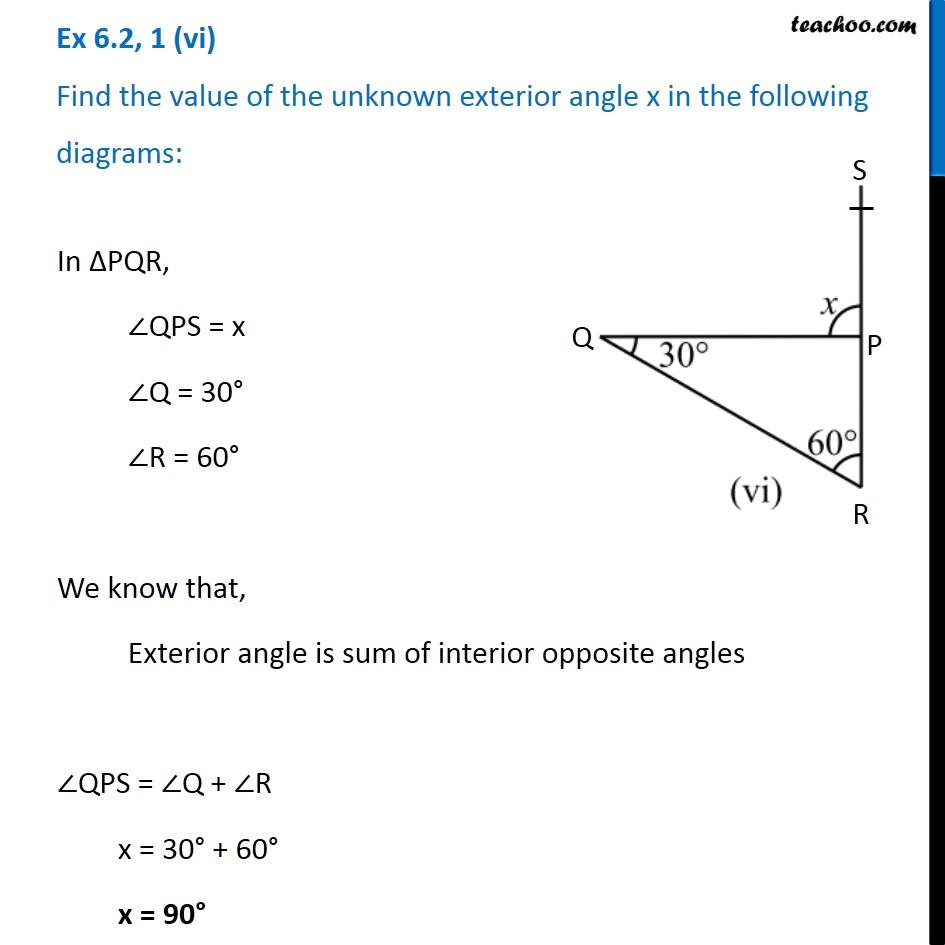1. Chapter 6 Class 7 Triangle and its Properties
2. Serial order wise
3. Ex 6.2

Transcript

Ex 6.2, 1 (vi) Find the value of the unknown exterior angle x in the following diagrams:In ∆PQR, ∠QPS = x ∠Q = 30° ∠R = 60° We know that, Exterior angle is sum of interior opposite angles ∠QPS = ∠Q + ∠R x = 30° + 60° x = 90°